Courses

# Test: Limits And Continuity : Intuitive Approach - 2

## 40 Questions MCQ Test Quantitative Aptitude for CA CPT | Test: Limits And Continuity : Intuitive Approach - 2

Description
This mock test of Test: Limits And Continuity : Intuitive Approach - 2 for CA Foundation helps you for every CA Foundation entrance exam. This contains 40 Multiple Choice Questions for CA Foundation Test: Limits And Continuity : Intuitive Approach - 2 (mcq) to study with solutions a complete question bank. The solved questions answers in this Test: Limits And Continuity : Intuitive Approach - 2 quiz give you a good mix of easy questions and tough questions. CA Foundation students definitely take this Test: Limits And Continuity : Intuitive Approach - 2 exercise for a better result in the exam. You can find other Test: Limits And Continuity : Intuitive Approach - 2 extra questions, long questions & short questions for CA Foundation on EduRev as well by searching above.
QUESTION: 1

Solution:
QUESTION: 2

Solution:
QUESTION: 3

###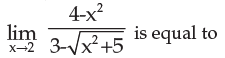Solution:
QUESTION: 4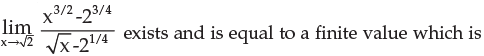Solution:
QUESTION: 5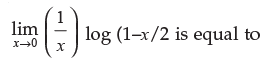Solution:
QUESTION: 6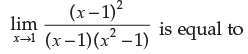Solution:
QUESTION: 7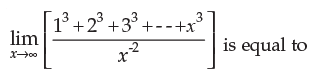Solution:
QUESTION: 8

If f(x) is an odd function then

Solution:
*Multiple options can be correct
QUESTION: 9

If f(x) and g(x) are two functions of x such that f(x) + g(x) = ex and f(x) – g(x) = e –x then

Solution:
*Multiple options can be correct
QUESTION: 10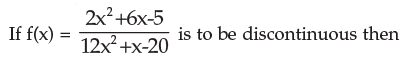Solution:
QUESTION: 11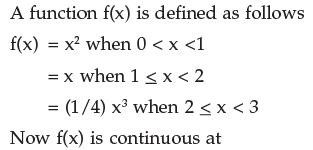Solution:
QUESTION: 12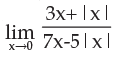Solution:
QUESTION: 13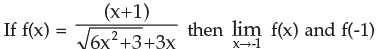Solution:
QUESTION: 14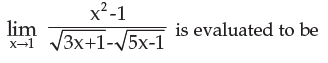Solution:
QUESTION: 15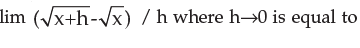Solution:
QUESTION: 16

Let f(x) = x when x >0

= 0 when x = 0

= – x when x < 0

Now f(x) is

Solution:
QUESTION: 17

If f(x) = 5+3x for x > 0 and f(x) = 5 – 3x for x < 0 then f(x) is

Solution:
QUESTION: 18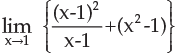Solution:
QUESTION: 19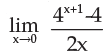Solution:
QUESTION: 20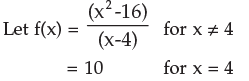Then the given function is not continuous for

Solution:
QUESTION: 21

A function f(x) is defined by f(x) = (x–2)+1 over all real values of x, now f(x) is

Solution:
QUESTION: 22

A function f(x) defined as follows f(x) = x+1 when x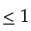= 3 – px when x > 1

The value of p for which f(x) is continuous at x = 1 is

Solution:
QUESTION: 23

A function f(x) is defined as follows :

f(x)= x when x < 1

= 1+x when x > 1

= 3/2 when x = 1

Then f(x) is

Solution:
QUESTION: 24

Let f(x) = x/|x|. Now f(x) is

Solution:
QUESTION: 25

f(x) = x–1 when x > 0

= – ½ when x = 0

= x + 1 when x < 0

f(x) is

Solution:
QUESTION: 26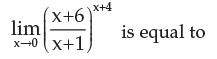Solution:
QUESTION: 27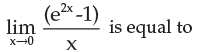Solution:
QUESTION: 28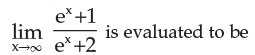Solution:
QUESTION: 29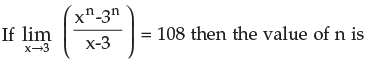Solution:
QUESTION: 30

f(x) = (x2 – 1) / (x3 – 1) is undefined at x = 1 the value of f(x) at x = 1 such that it is continuous at x = 1 is

Solution:
QUESTION: 31

f(x) = 2x – |x| is

Solution:
QUESTION: 32

If f(x) = 3, when x <2

f(x) = kx2, when x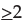is continuous at x = 2, then the value of k is

Solution:
QUESTION: 33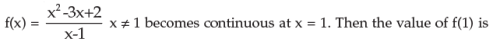Solution:
QUESTION: 34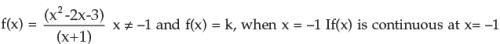The value of k will be

Solution:
QUESTION: 35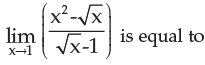Solution:
QUESTION: 36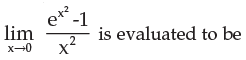Solution:
QUESTION: 37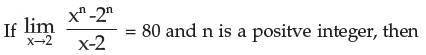Solution:
QUESTION: 38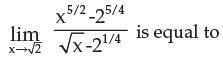Solution:
QUESTION: 39Solution:
QUESTION: 40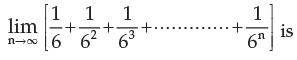Solution: